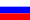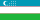Editorial board

Editor-in-Chief

Marapulets Yuriy Valentinovich – D. Sci. (Physical and Mathematical), Associate Professor, Deputy Director of the Institute of Space Physics Research and Radio Wave Propagation FEB RAS, Kamchatka region, with. Paratunka, Russia.

Scientific speciality:

Geophysics, geophysical methods of searches of minerals

Research interests: the study of acoustic radiation caused by geophysical processes; the development of acoustic methods of diagnosing natural environments, the development of specialized software.

e-mail: marpl@ikir.ruDeputy Editor

Parovik Roman Ivanovich – D. Sci. (Physi and Math.), Leading Researcher of physical processes modeling laboratory at the Institute of Space Physics Research and Radio Wave Propagation FEB RAS, Kamchatka region, v. Paratunka, Russia.

Scientific speciality:

Mathematical modeling, numerical methods and complexes of programs

Research interests: mathematical modeling hereditarity oscillatory systems, fractional calculus, numerical methods, software algorithms for the computer.

e-mail: parovik@krasec.ruExecutive Secretary

Feshchenko Lyubov Konstantinovna – PhD. (Phys. and Math.), Researcher of the Laboratory for Modeling Physical Processes of the Institute for Cosmophysical Research and Radio Wave Propagation, Far East Branch of the Russian Academy of Sciences.

Scientific speciality:

Mathematical modeling, numerical methods and program complexes

Research interests: mathematical modeling of dynamo dynamic processes for the magnetic fields of planets and stars, studies of the magnetic field in large-scale models of a two-mode dynamo with stochastic memory, numerical methods, computer program algorithms.

e-mail: feshenko.lk@yandex.ruMembers of the editorial board

Abirov Rustam Abdullaevich – D. Sci. (Phys. and Math.), Associate Professor, Head of the Laboratory “Deformation of Structural Materials under Complex Loading” at the Institute of Mechanics and Seismic Resistance of Structures named after M.T. Urazbayev Academy of Sciences of the Republic of Uzbekistan, Tashkent.

Scientific speciality:

Mechanics of a deformable solid

Research interests: theory of plasticity, mechanics of multiphase media, method of boundary elements.

e-mail: rustamabirov@mail.ruAshurov Ravshan Radzhabovich – D. Sci. (Phys. and Math.), Professor, Head of the Laboratory of Differential Equations and Their Applications of the Institute of Mathematics named after V.I. Romanovsky of the Academy of Sciences of Uzbekistan, Tashkent, Republic of Uzbekistan.

Scientific speciality:

Differential equations, dynamical systems and optimal control

Research interests: direct and inverse problems of mathematical physics, questions of their solvability, multiple trigonometric Fourier series.

e-mail: ashurovr@gmail.comVelmisov Petr Aleksandrovich – D. Sci. (Phys. and Math.), Professor, Head of the Department of Higher Mathematics, Ulyanovsk State Technical University.

Scientific specialities:

Mathematical modeling, numerical methods and program complexes.

Mechanics of liquid, gas and plasma.

Research interests: mathematical modeling (physical media; mechanical, technical and other systems and processes), aerohydromechanics, aerohydroelasticity, partial differential equations, stability.

Vodinchar Gleb Mikhailovich —  Ph. D. (Phys. and Math.), associate professor, head of the laboratory modeling of physical processes of the Institute of Space Physics Research and Radio Wave Propagation FEB RAS, Kamchatka region, v. Paratunka, Russia.

Scientific speciality:

Mathematical modeling, numerical methods and complexes of programs

Research interests: mathematical modeling of processes in the geosphere, stochastic processes, mathematical models of continuum mechanics, hydrodynamic dynamo model, non-local processes, computer software algorithms.

e-mail: gvodinchar@yandex.ruGoryushkin Aleksandr Petrovich – Ph. D. (Physical and Mathematical), Associate Professor, Department of Mathematics and Physics of the Kamchatka State University named after Vitus Bering, Petropavlovsk-Kamchatsky, Russia.

Scientific speciality:

Mathematical logic, algebra and number theory

Research interests: group theory, modern algebra, discrete mathematics, mathematical logic, number theory.

e-mail:as2021@mail.ruDzhamalov Sirozhiddin Zuhriddinovich – D. Sci. (Phys. and Math.), Associate Professor, Professor of the Department of Mathematics and Informatics of the Branch of the Russian State University of Oil and Gas (NRU) named after I.M. Gubkin in the city of Tashkent, Republic of Uzbeksitan.

Scientific speciality:

Differential equations, dynamical systems and optimal control

Mathematical physics

Research interests: partial differential equations, linear and nonlinear mixed-type equations of second and higher order, mixed-composite equations, local and nonlocal boundary value problems, inverse problems, theories of spectral operators.

e-mail: siroj63@mail.ruZikirov Obidzhan Salidzhanovich – D. Sci. (Physical and Mathematical), Dean of the Faculty of Mathematics of the National University of Uzbekistan named after Mirzo Ulugbek, Tashkent, Republic of Uzbekistan.

Scientific speciality:

Differential equations, dynamical systems, and optimal control

Research Interests: partial differential equations; local and nonlocal boundary value problems for nonclassical equations of mathematical physics; and composite equations of mixed-composite type.

e-mail: zikirov@yandex.ruNagorskiy Petr Mikhaylovich – D. Sci. (Phys. and Math.), professor, leading researcher of the Laboratory of Physics of the Institute of monitoring of climatic systems of climatic and ecological systems SB RAS, Tomsk, Russia.

Scientific speciality:

Environmental protection and rational use of natural resources

Research interests: scientific and methodological support of the regional climate and environmental monitoring; study spatial and temporal variability of meteorological variables fields in the Siberian region and the identification of the physical processes that underlie this variability; Multivariate analysis and valuation of ecosystem and climate change.

e-mail: npm_sta@mail.ruPortnyagin Nikolay Nikolaevich – D. Sci. (Tech.), Professor, Department of Theoretical Electrical Engineering and electrification of the oil and gas industry of the Russian State University named after Gubkin, Moscow, Russia.

Scientific speciality:

Automation and management of technological processes and manufactures (on areas)

Research interests: the creation of means and methods of diagnostics of electrical systems, electrical systems modeling elements, models and algorithms of automation power supply.

e-mail: pornic1@yandex.ruPskhu Arsen Vladimirovich – D. Sci. (Phys. and Math.), Professor, Director, Institute of Applied Mathematics and Automation, Nalchik, Kabardino-Balkaria, Russia.

Scientific speciality:

Differential equations, dynamical systems, and optimal control

Research interests: differential equations in partial derivatives, ordinary differential equations, fractional calculus, the existence and uniqueness of nonlocal boundary value problems of mathematical physics.

e-mail: pskhu@list.ruRakhmonov Zafar Ravshanovich – D. Sci. (Phys. and Math.), Dean of the Faculty of Applied Mathematics and Intellectual Technologies of the National University of Uzbekistan, Tashkent, Uzbekistan.

Scientific speciality:

Mathematical modeling, numerical methods and program complexes

Research interests: mathematical modeling of nonlinear processes, asymptotic theory of nonlinear ODEs and differential equations in partial derivatives, computational methods of nonlinear boundary value problems.

e-mail:zraxmonov@inbox.ruRekhviashvili Sergo Shotovich – D. Sci. (Physical and Mathematical), Professor, Deputy Director for Science, Institute of Applied Mathematics and Automation, Nalchik, Republic of Kabardino-Balkaria, Russia.

Scientific speciality:

Experimental Physics Devices and methods

Research interests: physics of condensed state, the application of the theory of fractals and fractional integro-differentiation, size effects, probe nanotechnology.

e-mail: rsergo@mail.ruSerbina Lyudmila Ivanovna – D. Sci. (Phys. and Math.), Professor, Honorary Worker of the Higher Professional Education of the Russian Federation, Professor of the Department of Mathematics and Informatics of the State Pedagogical Institute in Stavropol.

Scientific specialty:

Mathematical modeling, numerical methods and program complexes

Research interests: application of operators of fractional integro-differentiation in mathematical modeling of nonlinear dynamic processes of mass transfer with fractal space-time structure; local and nonlocal boundary value problems for loaded equations of basic and mixed types and their practical application in the study of the anomalous regime of nonlinear filtration of homogeneous liquids in saturated capillary-porous media.

e-mail: lserbina@mail.ruSmirnov Sergey Eduardovich – D. Sci. (Phys. and Math.), Leading Researcher of the Laboratory of Systems Analysis of the Institute of Cosmophysical Research and Radio Wave Propagation, Far East Branch of the Russian Academy of Sciences.

Scientific speciality:

Physics of the atmosphere and hydrosphere

Research interests: the Earth’s electric field, solar activity, earthquake precursors

e-mail: sergey@ikir.ruHayotov Abdullo Rakhmonovich – D. Sci. ( Phys. and Math.), Head of the Computational Mathematics Laboratory of the Institute of Mathematics named after V.I. Romanovsky Academy of Sciences of Uzbekistan, Tashkent, Republic of Uzbekistan.

Scientific speciality:

Computational Mathematics

Research interests: theory of cubatures and quadrature formulas, approximation and interpolation, theory of splines, numerical solution of differential and integral equations, computed tomography.

e-mail: hayotov@mail.ruShibzukhov Zaur Mukhadinovich – D. Sci. (Phys. and Math.), professor of theoretical computer science and discrete mathematics at Moscow State Pedagogical University, Moscow, Russia.

Scientific speciality:

Theoretical foundations of computer science

Research interests: machine learning, neural networks, theoretical foundations of informatics, neuroinformatics, the theory of pattern recognition

e-mail: szport@gmail.comYakovleva Valentina Stanislavovna  – D. Sci. (Tech.), Professor, Department of Applied Physics of the National Research Tomsk Polytechnic University, Tomsk, Russia.

Scientific speciality:

Devices and methods of monitoring an environment, substances, materials and products.

Research interests: investigation of mass transfer of radon in the system “lithosphere-atmosphere” and its impact on the ground layer of the atmosphere, the development of instruments and methods of control of radon in the ground layer of the atmosphere, numerical methods, software algorithms for the computer.

e-mail: vsjakovleva@tpu.ruYakovleva Tat’yana Petrovna – Ph.D. (Ped.), assistant professor of mathematics and physics of the Vitus Bering Kamchatka State University, Petropavlovsk-Kamchatsky, Russia.

Scientific speciality:

Theory and methods of professional education

Research interests: the theory and methods of teaching mathematics in vocational education.

e-mail: yakovleva@inbox.ru